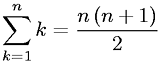Equations > Calculus > Sequences and Series > Arithmetic Series - Sequential Integers

### Arithmetic Series - Sequential IntegersLatex Code:

MathML Code:

 $\sum _{k=1}^{n}k=\fracn\left(n+1\right)2$

MathType 5.0: